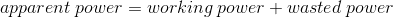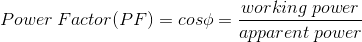##### Question

In: Electrical Engineering

# power factor improvement methods for the energy conservation in drives

power factor improvement methods for the energy conservation in drives

## Solutions

##### Expert Solution

Power Factor is defined as the ratio of working or useful power to the apparent power.More the Power Factor, more the device is energy efficient.hence, these are the power factor improvement methods for the energy conservation in drives.

## Related Solutions

##### What are the methods of energy conservation in electrical drives
What are the methods of energy conservation in electrical drives
##### Discuss the disadvantages of having low power factor and discuss the various methods of power factor...
Discuss the disadvantages of having low power factor and discuss the various methods of power factor improvement.
##### Energy conservation law
Just before striking the ground, a 2.0kg mass has 400J of kinetic energy. If friction can be ignored, from what height was it dropped?(g = 9.8m/s2)
##### A brief summary about: Newton’s laws Friction and circular movement Work-energy(kinetic and potential)-power-and conservation of energy...
A brief summary about: Newton’s laws Friction and circular movement Work-energy(kinetic and potential)-power-and conservation of energy Fluid( pascal’s law,buyoant force,Archimedes principle, fluid flow and viscosity and bernoulli’s equation)
##### 2. Describe Kinetic Energy and Potential Energy. Describe the Law on Conservation of Mechanical Energy. If...
2. Describe Kinetic Energy and Potential Energy. Describe the Law on Conservation of Mechanical Energy. If an object that is thrown perpendicular to the ground by neglecting the friction is required to go up to 125 m height, what should be the initial velocity of the object? Take the acceleration of gravity 10 m / s and assume that the mass of the object does not change.
##### - In your own words, explain potential energy, kinetic energy, conservation of energy and examples in...
- In your own words, explain potential energy, kinetic energy, conservation of energy and examples in daily life. - In your own words, explain the definition of work in Physics. - In your own words, explain Hooke's Law and elastic potential energy.
##### Power Factor Code Arduino Develop a Power factor Program in C and upload it into your...
Power Factor Code Arduino Develop a Power factor Program in C and upload it into your Arduino #include <LiquidCrystal.h> LiquidCrystal lcd(12, 11, 5, 4, 3, 2);
##### 3. a)What is kinetic energy? b) What is potential energy? c) What is the law of conservation of energy
3. a)What is kinetic energy? b) What is potential energy? c) What is the law of conservation of energy 4. a)What is momentum? b) What is the law of conservation of momentum?
##### Could some give a deep descripition about the power factor. 1. How the power factor will...
Could some give a deep descripition about the power factor. 1. How the power factor will be less? 2.What should we do inorder to increase the power factor? 3.How do we maintain a good power factor in a 3 phase motor without using the capacitor?
##### Explain conservation of mechanical energy (including formula) in terms of potential and kinetic energy using an...
Explain conservation of mechanical energy (including formula) in terms of potential and kinetic energy using an example in your sport